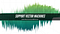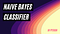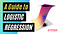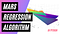# SVM Classifier and RBF Kernel — How to Make Better Models in Python

## A complete explanation of the inner workings of Support Vector Machines (SVM) and Radial Basis Function (RBF) kernelSVM with RBF kernel and high gamma. See how it was created in the Python section at the end of this story. Image by author.

# Intro

It is essential to understand how different Machine Learning algorithms work to succeed in your Data Science projects.

I have written this story as part of the series that dives into each ML algorithm explaining its mechanics, supplemented by Python code examples and intuitive visualizations.

# The story covers the following topics:

• The category of algorithms that SVM classification belongs to
• An explanation of how the algorithm works
• What are kernels, and how are they used in SVM?
• A closer look into RBF kernel with Python examples and graphs

# What category of algorithms does Support Vector Machines classification belong to?

Support Vector Machines (SVMs) are most frequently used for solving classification problems, which fall under the supervised machine learning category. …

# Naive Bayes Classifier — How to Successfully Use It in Python?

## A detailed explanation of the theory behind the algorithm together with 6 Python examplesNaive Bayes Model Decision Boundaries. Image by author. (See section 5 for how this graph was made).

# Preface

Just so you know what you are getting into, this is a long story that contains a mathematical explanation of the Naive Bayes classifier with 6 different Python examples. Please take a look at the list of topics below and feel free to jump to the most interesting sections for you.

# Intro

Machine Learning is making huge leaps forward, with an increasing number of algorithms enabling us to solve complex real-world problems.

This story is part of a deep dive series explaining the mechanics of Machine Learning algorithms. …

# Logistic Regression in Python— A Helpful Guide to How It Works

## A detailed explanation of the algorithm together with useful examples on how to build a model in PythonLogistic Regression. Image by author. (See how this graph was made in the Python section below)

# Preface

Just so you know what you are getting into, this is a long story that contains a visual and a mathematical explanation of logistic regression with 4 different Python examples. Please take a look at the list of topics below and feel free to jump to the sections that you are most interested in.

# Intro

Machine Learning is making huge leaps forward, with an increasing number of algorithms enabling us to solve complex real-world problems.

This story is part of a deep dive series explaining the mechanics of Machine Learning algorithms. …

# Intro

Machine Learning is making huge leaps forward, with an increasing number of algorithms enabling us to solve complex real-world problems.

This story is part of a deep dive series explaining the mechanics of Machine Learning algorithms. In addition to giving you an understanding of how ML algorithms work, it also provides you with Python examples to build your own ML models.

# This story covers the following topics:

• The category of algorithms that SVR belongs to
• An intuitive explanation of how SVR works
• A few examples of how to build SVR models in Python

# What category of algorithms does Support Vector Regression belong to?

While you may not be familiar with SVR, chances are you have previously heard about Support Vector Machines (SVM). SVMs are most frequently used for solving classification problems, which fall under the supervised machine learning category. …

# Intro

Machine Learning is making huge leaps forward, with an increasing number of algorithms enabling us to solve complex real-world problems.

This story is part of a deep dive series explaining the mechanics of Machine Learning algorithms. In addition to giving you an understanding of how ML algorithms work, it also provides you with Python examples to build your own ML models.

# This story covers the following topics:

• What category of algorithms does LOWESS belong to?
• How does Locally Weighted Scatterplot Smoothing work?
• How can I use LOWESS to identify patterns and predict new data in Python?

# What category of algorithms does LOWESS belong to?

Locally Weighted Scatterplot Smoothing sits within the family of regression algorithms under the umbrella of Supervised Learning. This means that you need a set of labeled data with a numerical target variable to train your model. …

# MARS: Multivariate Adaptive Regression Splines — How to Improve on Linear Regression?

## A visual explanation of the MARS algorithm with Python examples and comparison to linear regressionModel prediction comparison between MARS and Linear Regression. Image by author.

# Intro

Machine Learning is making huge leaps forward, with an increasing number of algorithms enabling us to solve complex real-world problems.

This story is part of a deep dive series explaining the mechanics of Machine Learning algorithms. In addition to giving you an understanding of how ML algorithms work, it also provides you with Python examples to build your own ML models.

Before we dive into the specifics of MARS, I assume that you are already familiar with Linear Regression. If you would like a refresher on the topic, feel free to explore my linear regression story:

# This story covers the following topics:

• What category of algorithms does MARS belong to? …

# Intro

Machine Learning is making huge leaps forward, with an increasing number of algorithms available so we can solve complex real-world problems.

This story is part of a deep dive series explaining the mechanics of Machine Learning algorithms. In addition to giving you an understanding of how ML algorithms work, it will also provide you Python examples so you can use them to build your own ML models.

# What is covered in this story?

• What category of algorithms does linear regression belong to?
• What problems can be solved using linear regression?
• How does the linear regression algorithm work?
• How can I use linear regression to build a model in Python? …

# How to Get Medium Story Stats with 3 Lines of Python Code

## A step by step guide in how to extract and read a JSON file containing your stats into a Pandas DatFrame

Suppose you are a person in the Data Science community who publishes stories on Medium. In that case, there is no doubt you have considered doing some additional analytics using the stats of your stories.

While I came across other stories that explain how to extract Medium stats, they required a significant amount of coding using “Beautiful Soup” to parse an HTML file and find relevant information by looking for the right tags.

My proposed solution is much simpler since it only needs to read a JSON file and convert it to Pandas DataFrame. …

# What I Learned About Luck From Running 10,000 Simulations

## Analyzing the balance between luck and skill through the lens of probabilities

The question about the importance of luck has been on my mind for a long time. Whether it is getting into the university that I wanted, finding a job, getting a promotion, or winning a sports fantasy game. As I am a person who analyzes everything, I find myself constantly thinking about the underlying drivers behind successes and failures.

Most recently, I pondered about luck after reviewing the stats for my Medium stories. Since I am a new writer, I still have a lot to learn, but I expect to get better. …

# How to Create a 3D Model of the Solar System Using Plotly in Python

## Step by step guide in how to use Plotly 3D graphs

There is a large selection of graphing libraries in Python that enables you to plot all the major types of charts so you can visualize your data. However, have you ever considered using them for something a bit more sophisticated? Put Matplolib and Bokeh aside and dive into the wonderful world of 3D and animated graphs that can be created using Plotly.

I have no association with Plotly but I have been using their library in Python for a while now and I find it very useful. Hence, I decided to explore it deeper.

Here, I am using a combination of Plotly Scatter3D and Plotly Surface plots to create an entire Solar system inside one graph. In this story, I will take you through the Python code that will enable you to recreate it yourself. …